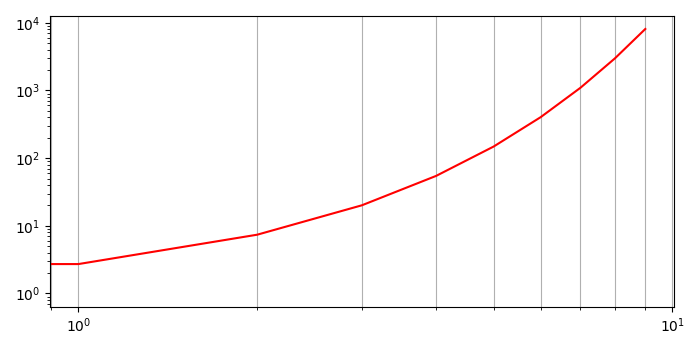# How do I show logarithmically spaced grid lines at all ticks on a log-log plot using Matplotlib?

MatplotlibServer Side ProgrammingProgramming

To show logarithmically spaced grid lines at all ticks on a log-log plot using matplotlib, we can take the following steps−

• Create data points for x and y using numpy.
• Using loglog method, make a plot with log scaling on both the X and Y axis.
• Use grid() method, lay out a grid in the current line style. Supply the list of x an y positions.
• To display the figure, use show() method.

## Example

import numpy as np
from matplotlib import pyplot as plt
plt.rcParams["figure.figsize"] = [7.00, 3.50]
plt.rcParams["figure.autolayout"] = True
x = np.arange(0, 10, 1)
y = np.exp(x)
plt.loglog(x, y, c='r')
plt.grid(True, which="both", axis='x')
plt.show()

## Output